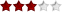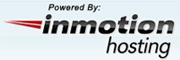Categories

﻿
1

Making Bar Charts using ASPThis is an ASP based tutorial that helps you to generate static and dynamic bar charts easily. This tutorial provides the source code for creating these charts which is useful for all the programmers.

Cost: Free

Simple Graphs With ASPThis tip helps you to create simple line graphs without using any COM objects. You can change the color, height,and width of the bars easily. This code is written in ASP.

Cost: Free

ASP Bar ChartsThis easy to use tutorial has an useful script that contains the code for generating bar charts. This script is written in ASP. You can easily change the attributes of the bar charts generated by this script.

Cost: Free

Graphs from a databaseThis is an useful bar graph tip that provides you the source code for generating bar graphs. This code can be used on any form of data base.

Cost: Free

Version: 1.2

ASP Cool ChartThis tutorial gives the source code that helps you to create the bar charts. You simply enter the values to draw the bar charts. This code is written in ASP. This is an useful tutorial for the application developers.

Cost: Free

Horizontal Percentage GaugeThis is an useful tip that helps users to create a horitantal percentage gauge. This graphical tool is useful to represent the test marks in the quiz section. This tip provides the example source code for the users.

Cost: Free

HTML charting in ASP - stacked bar chartThis tutorial helps users to create the stacked html bar chart with reference to URL from each data point and data point title description. This tutorial shows the sample code for the users.

Cost: Free

HTML charting in ASP - basicsThis chart tip helps you to create the charts using html directly on the web pages. You do not need to use the gif, jpg files and server objects to draw the chart.

Cost: Free

Bargraph in ASPThis is a graph tutorial that shows an easy way to generate bar graphs. These bar graphs are created by providing the input data.

Cost: Free

Haneng ChartsThis tutorial explains you about how to create interactive bar graphs. You can generate the bar graphs on your application by integrating the source code provided by this tutorial. This is an easy to follow tutorial.

Cost: Free

1

Need Scripts Message

We at Need Scripts, help You For Free, You can help us to serve you even better!General Web Hosting

Platform Based Hosting

Programmer's Hosting

Special Need's Hosting

Web Hosting Resources